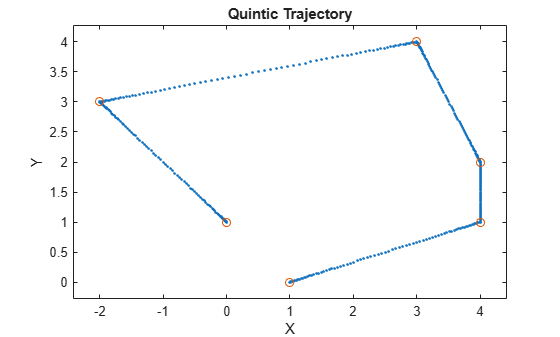quinticpolytraj

Generate fifth-order trajectories

Description

example

[q,qd,qdd,pp] = quinticpolytraj(wayPoints,timePoints,tSamples) generates a fifth-order polynomial that achieves a given set of input waypoints with corresponding time points. The function outputs positions, velocities, and accelerations at the given time samples, tSamples. The function also returns the piecewise polynomial pp form of the polynomial trajectory with respect to time.

[q,qd,qdd,pp] = quinticpolytraj(___,Name,Value) specifies additional parameters as Name,Value pair arguments using any combination of the previous syntaxes.

Examples

collapse all

Use the quinticpolytraj function with a given set of 2-D xy waypoints. Time points for the waypoints are also given.

wpts = [1 4 4 3 -2 0; 0 1 2 4 3 1];
tpts = 0:5;

Specify a time vector for sampling the trajectory. Sample at a smaller interval than the specified time points.

tvec = 0:0.01:5;

Compute the quintic trajectory. The function outputs the trajectory positions (q), velocity (qd), acceleration (qdd), and polynomial coefficients (pp) of the quintic polynomial.

[q, qd, qdd, pp] = quinticpolytraj(wpts, tpts, tvec);

Plot the quintic trajectories for the x- and y-positions. Compare the trjactory with each waypoint.

plot(tvec, q)
hold all
plot(tpts, wpts, 'x')
xlabel('t')
ylabel('Positions')
legend('X-positions','Y-positions')
hold offYou can also verify the actual positions in the 2-D plane. Plot the separate rows of the q vector and the waypoints as x- and y- positions.

figure
plot(q(1,:),q(2,:),'.b',wpts(1,:),wpts(2,:),'or')
xlabel('X')
ylabel('Y')Input Arguments

collapse all

Points for waypoints of trajectory, specified as an n-by-p matrix, where n is the dimension of the trajectory and p is the number of waypoints.

Example: [1 4 4 3 -2 0; 0 1 2 4 3 1]

Data Types: single | double

Time points for waypoints of trajectory, specified as a p-element vector.

Example: [0 2 4 5 8 10]

Data Types: single | double

Time samples for the trajectory, specified as an m-element vector. The output position, q, velocity, qd, and accelerations, qdd, are sampled at these time intervals.

Example: 0:0.01:10

Data Types: single | double

Name-Value Arguments

Specify optional comma-separated pairs of Name,Value arguments. Name is the argument name and Value is the corresponding value. Name must appear inside quotes. You can specify several name and value pair arguments in any order as Name1,Value1,...,NameN,ValueN.

Example: 'VelocityBoundaryCondition',[1 0 -1 -1 0 0; 1 1 1 -1 -1 -1]

Velocity boundary conditions for each waypoint, specified as the comma-separated pair consisting of 'VelocityBoundaryCondition' and an n-by-p matrix. Each row corresponds to the velocity at all of p waypoints for the respective variable in the trajectory.

Example: [1 0 -1 -1 0 0; 1 1 1 -1 -1 -1]

Data Types: single | double

Acceleration boundary conditions for each waypoint, specified as the comma-separated pair consisting of 'VelocityBoundaryCondition' and an n-by-p matrix. Each row corresponds to the acceleration at all of p waypoints for the respective variable in the trajectory.

Example: [1 0 -1 -1 0 0; 1 1 1 -1 -1 -1]

Data Types: single | double

Output Arguments

collapse all

Positions of the trajectory at the given time samples in tSamples, returned as an m-element vector, where m is the length of tSamples.

Data Types: single | double

Velocities of the trajectory at the given time samples in tSamples, returned as a vector.

Data Types: single | double

Accelerations of the trajectory at the given time samples in tSamples, returned as a vector.

Data Types: single | double

Piecewise-polynomial, returned as a structure that defines the polynomial for each section of the piecewise trajectory. You can build your own piecewise polynomials using mkpp, or evaluate the polynomial at specified times using ppval. The structure contains the fields:

• form: 'pp'.

• breaks: p-element vector of times when the piecewise trajectory changes forms. p is the number of waypoints.

• coefs: n(p–1)-by-order matrix for the coefficients for the polynomials. n(p–1) is the dimension of the trajectory times the number of pieces. Each set of n rows defines the coefficients for the polynomial that described each variable trajectory.

• pieces: p–1. The number of breaks minus 1.

• order: Degree of the polynomial + 1. For example, cubic polynomials have an order of 4.

• dim: n. The dimension of the control point positions.